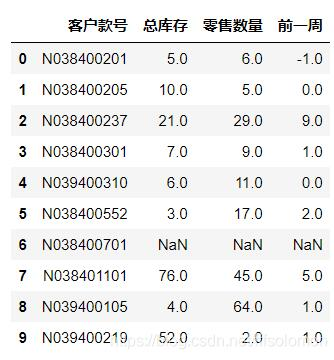python 使用xlsxwriter循環向excel中插入數據和圖片的操作import xlsxwriter
import os
#以空字符填充缺失值，不然寫入數據會報錯
data.fillna('',inplace=True)
#創建一個新Excel文件並添加一個工作表。
workbook = xlsxwriter.Workbook('images.xlsx')
# # 加寬第2列，,根據圖片縮放大小進行調整。
worksheet.set_column('B:B', 20)
worksheet.set_column('D:D', 20)
worksheet.set_column('F:F', 20)
worksheet.set_column('H:H', 20)
##寫入數據和圖片
for i in range(len(data)):
for j in range(4):
worksheet.write(i//5*16+j+12,i%5*2 ,['客戶款號','總庫存','零售數量','前一周'][j])
worksheet.write(i//5*16+j+12,i%5*2+1 ,data.iloc[i,0::].values[j])
#插入圖片，insert_image(位置行，位置列,文件名,縮放比例)
if data.iloc[i,0::].values+'.jpg' not in os.listdir('.\img\\'):
print(i,'找不到',data.iloc[i,0::].values+'.jpg')
else:
worksheet.insert_image(i//5*16,i%5*2+1,'img/'+data.iloc[i,0::].values+'.jpg',{'x_scale': 0.1, 'y_scale': 0.12})
print(i,'寫入成功!')
i+=1
workbook.close()
0 寫入成功!
1 寫入成功!
2 找不到 N038400237.jpg
3 找不到 N038400301.jpg
4 找不到 N039400310.jpg
5 找不到 N038400552.jpg
6 寫入成功!
7 找不到 N038401101.jpg
8 找不到 N039400105.jpg
9 找不到 N039400219.jpg（2）、操作xlsx格式的表格文件：

*如果用操作xls的方法去寫入xlsx文件，會導致文件損壞無法打開；反之一樣。

（一）、操作xls格式表格

1、讀取excel表格數據

import xlrd  #從excle裡讀數據
import xlwt  #創建新的表格寫入數據
import xlutils #往已有表格中追加數據

class IOExcel(object):
def __init__(self,file):
self.file = file

def get_sheet(self,sheetname):
excelfile=xlrd.open_workbook(self.file)
self.sheet = excelfile.sheet_by_name(sheetname)
return self.sheet
#獲取第*行的數據
def get_rowData(self,row):
cols = self.sheet.ncols  #獲取sheet頁有多少列
Cells = []
for i in range(0,cols):
Cells.append(self.sheet.cell(row,i).value)
return Cells

2、創建表格寫入數據

def write_excel(self,sheet_name, value):
index = len(value) # 獲取需要寫入數據的行數
workbook = xlwt.Workbook() # 新建一個工作簿
for i in range(0, index):
for j in range(0, len(value[i])):
sheet.write(i, j, value[i][j])   #向表格中寫入數據（對應的行和列）
workbook.save(self.file)   # 保存工作簿

3、向已存在表格中追加數據

def write_excel_xls_append(self,value):
index = len(value) # 獲取需要寫入數據的行數
workbook = xlrd.open_workbook(self.file) # 打開工作簿
sheets = workbook.sheet_names() # 獲取工作簿中的所有表格
worksheet = workbook.sheet_by_name(sheets) # 獲取工作簿中所有表格中的的第一個表格
rows_old = worksheet.nrows # 獲取表格中已存在的數據的行數
new_workbook = copy(workbook) # 將xlrd對象拷貝轉化為xlwt對象
new_worksheet = new_workbook.get_sheet(0) # 獲取轉化後工作簿中的第一個表格
for i in range(0, index):
for j in range(0, len(value[i])):
new_worksheet.write(i+rows_old, j, value[i][j]) # 追加寫入數據，註意是從i+rows_old行開始寫入
new_workbook.save(self.file) # 保存工作簿

（二）、操作xlsx格式表格

wb = openpyxl.Workbook()  #創建一個新的excel
we = wb.create_sheet('第二頁',0) #修改sheet頁的名字；設置插入sheet頁的位置，默認在上一頁後面（ 初始創建的excel是隻有一個默認sheet頁的，所以設置位置的值大於1效果都一樣，都是在默認sheet頁的後面接著）
# we.title = '你好' # 修改sheet頁的名字
#操作單元格
we['A1']=123124
we['B2']='你好'
print(we.cell(1,2,'123123').value)  #設置cell的行號和列號和值，返回cell的值
wb.save('C:\\Users\\t_ful\\PycharmProjects\\test\\element\\t.xlsx')  #保存表格